Earth Sciences
Volume 5, Issue 4, August 2016, Pages: 56-61

Determination of Mine Location by Using Edge Detection Methods: A Case Study of Sivas-Divrigi

Fikret Dogru1, Oya Pamukcu1, Cagatay Pamukcu2, *

1Department of Geophysical Engineering, Dokuz Eylul University, Izmir, Turkey

2Department of Mining Engineering, Dokuz Eylul University, Izmir, Turkey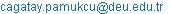(C. Pamukcu)

*Corresponding author

Fikret Dogru, Oya Pamukcu, Cagatay Pamukcu. Determination of Mine Location by Using Edge Detection Methods: A Case Study of Sivas-Divrigi. Earth Sciences. Vol. 5, No. 4, 2016, pp. 56-61. doi: 10.11648/j.earth.20160504.12

Received: June 28, 2016; Accepted: July 16, 2016; Published: September 13, 2016

Abstract: Edge detection and edge enhancement techniques play a crucial role in interpreting potential field data. There have been various edge detection applications to magnetic data in order to determine the edges of subsurface structures in geophysics. The edge detection methods comprise analytic signal, total horizontal derivative, theta angle, tilt angle. In synthetic case, two prism model used and the edge detection filters were applied on synthetic data successfully. In field study, the magnetic anomaly of Sivas-Divrigi mining area was selected because of importance of this area in terms of high-grade iron ore reserves. The mentioned method was applied on field data and the location of iron ore was revealed. As a conclusion, tilt angle and theta map methods gave a good agreement for determination of the structure location in both synthetic and field studies. In addition, tilt angle of the vertical derivative was studied both in theoretical and field applications. It was seen that residual information could be obtained in case tilt angle was taken following the vertical derivation.

Keywords: Magnetic, Geophysics, Mining, Edge Techniques

Contents

1. Introduction

Gravity and magnetic anomalies are essential ranging from geophysical approaches to geologic mapping . Determination of the burried structure is one of the most important stages in the modeling of gravity anomalies. Accurate determination of source body coordinates is the main purpose for interpretation and therefore enhanced methods are acquiring an increasing revival in data interpretation [2-3]. There are various procedures that have been engaged to attain edge detection, for example, horizontal gradient method (THDR), analytic signal (AS), tilt angle (TA), theta map (TM) and etc. . When previous studies conducted about the usage of those methods are investigated; Salem et. al.  evaluated the magnetic anomaly by using the derivatives of the tilt angle. Beiki  used the analytical signal operator in order to determine the structure boundaries out of gravity gradient components. Roy  applied the method of tilt angle to 2D magnetic profile data that were obtained from normal and inverse fault models. Cooper  used the analytical signal and tilt angle method in the detection of structure boundaries in aeromagnatic data. Hidalgo and Gato  used the tilt angle and analytical signal operators in order to compare it with a new method proposed by themselves. On the other hand, Yan et. al.  proposed analytical signal tilt angle method developed as a novel edge detection operator. Besides, Yan et. al.  also usd the theta map method and compared the consequences with the anlytical signal operator.

Within the scope of this study; firstly THDR, AS, TA, TM methods, which are intended to determine magnetic bodies, were employed by using synthetic models that comprise magnetic anomalies. Then, these methods were applied to the real magnetic anomalies that exist at the mine site located in Sivas-Divrigi (Figure 1).

In Divrigi region, there are generally ophiolitic, sedimentary, granitoitic, metamorphic and volcanic rocks. Three main tectonic units exist in the vicinity of Sivas, in which the study area is also located. These tectonic units are represented from north to the south as Pontide Tectonic Zone, North Anatolia Ophiolite Zone and Taurus Tectonic Zone, respectively. The metamorphic massifs in the region are the equivalences of platform-type carbonates which have gone through metamorphism. Tectonic units are overlain in discordance by an overburden strata having a Quarternary geologic age .

When the general geology of the region is investigated, the ore is embedded within the contact of granitic rocks and serpantinized ultrabasic rocks, called felses. Although the waste rock is majorly composed of felses and granitic rocks, it is sometimes comprised of serpantinite and silicified-carbonated ultrabasic rocks. Within the ore itself, occasional bands of fels, serpantinite and silicified-carbonated rock residues are observed. The ore is mainly magnetite, hematite and limonite but secondarily, pyrite and chalcopyrite veinlets are also encountered. The mean grade value of the ore body is nearly 55% .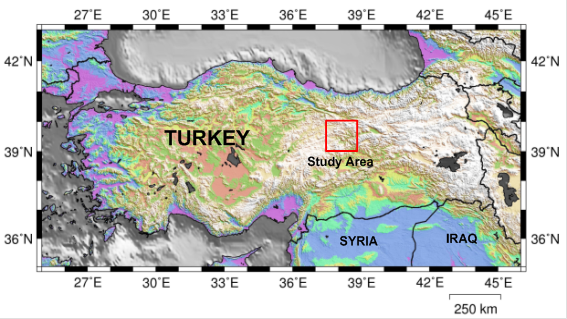Figure 1. The location map of study area.

THDR method shows minimum values within the body in the synthetic study while it yields maximum values on the boundaries. With respect to AS results, the amplitude of the anomaly seems to increase but no relevant information was reached about the boundaries of the body. However, regarding the results of tilt angle and theta map, maximum values were observed within the body whereas minimum values were observed on the edges in the tilt angle. Similarly, maximum values were monitored within the structure while minimum values were seen along the edges in theta map. The field studies were also found consistent with the same scenarios mentioned in synthetic models. In regards to tilt angle, the decrease on the boundaries happen to be linear whereas it comprises a sequence of maximum-minimum trend in theta map.

2. Methods

A number of methods have been proposed to make subtle anomalies more visible. The first filter developed for this purpose was the tilt angle , which is the ratio of the vertical derivative to the absolute value of the horizontal derivative of the potential field. The tilt angle is given bywhereP is the potential anomaly and THDR is the total horizontal derivative . The tilt angle produces a zero value over the source edges.

Wijns et al (2005)  introduced the theta map (θ), which is the normalization of the THDR by the AS, is given by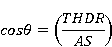andAS is the analytic signal for the 3D case . The theta map delineates model edges well, but the response of deeper bodies is diffused; consequently, it does not produce the expected sharp gradient over the edges.

3. Applications

In this stage, two prism models anomaly was utilized for theoretical studies. Synthetic anomalies were obtained by using Potensoft program which is based on MATLAB  and the 3D view of model was given in Figure 2. After that, edge detection algorithms were carried out on both noise-free and noise-corrupted theoretical data.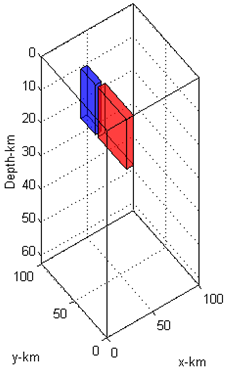Figure 2. Model of two prism (Depths are 10, 25 km, Magnetisation intensity 0.1 A/m, Inclination 90°, declination 0°).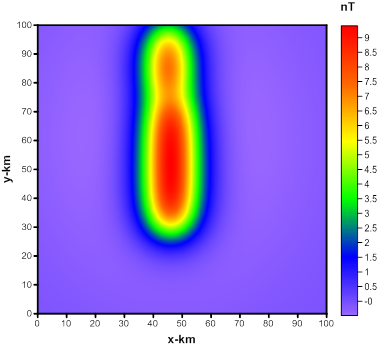Figure 3. Synthetic anomaly of two prism model.

Figure 4. Results of edge detection filters: a) THDR, b) AS, c) Tilt, d) Theta map.

Within the field studies; THDR, AS, TA and TM methods were applied to magnetic map depicted in Figure 5, which belongs to a iron ore deposit located in Sivas-Divrigi. The obtained results are shown in Figure 6.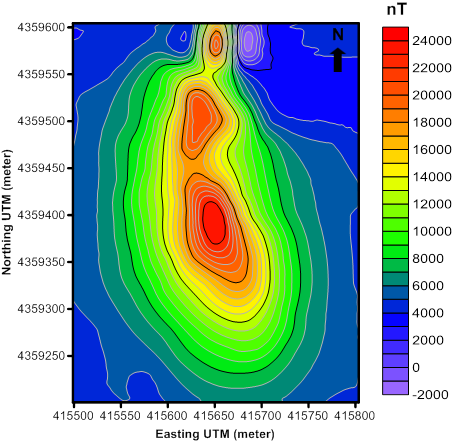Figure 5. Magnetic anomaly of Divrigi mining field.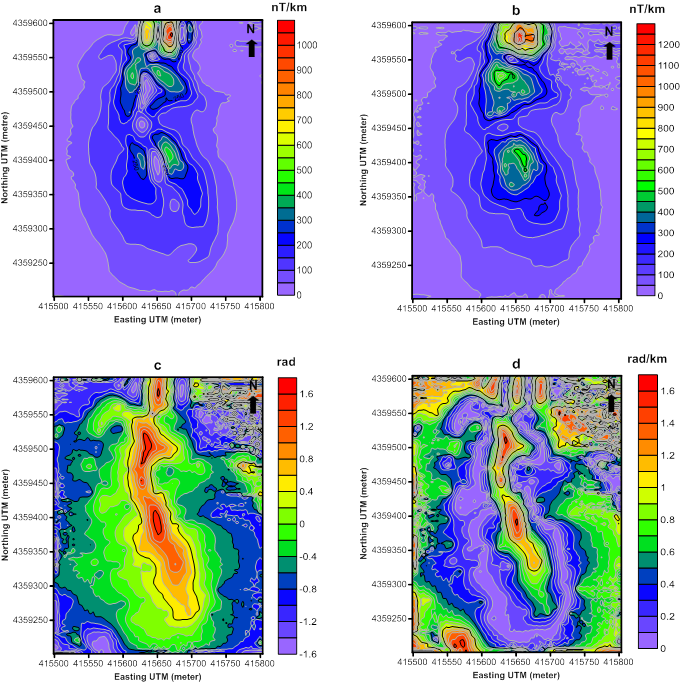Figure 6. Results of edge detection filters on field study: a) THDR, b) AS, c) Tilt, d) Theta map.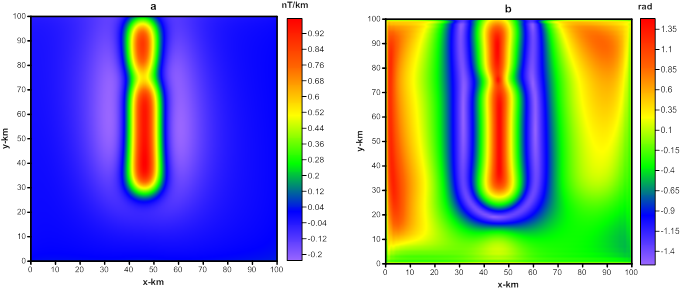Figure 7. Results of edge detection filters on synthetic study: a) z derivative of magnetic anomaly, b) tilt angle of z derivative of magnetic anomaly.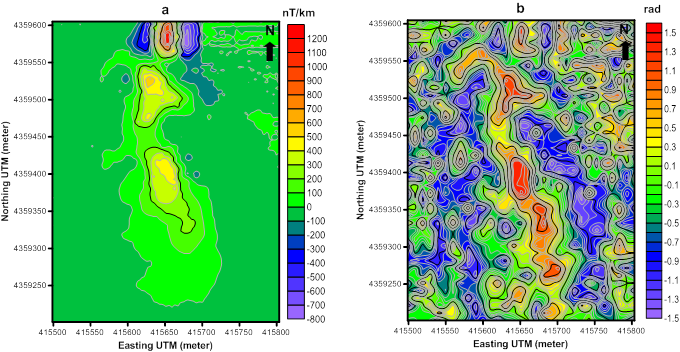Figure 8. Results of edge detection filters on field study: a) z derivative of magnetic anomaly, b) tilt angle of z derivative of magnetic anomaly.

By using the approach proposed in a recent study conducted by Oruc  in 2010, vertical derivatives of theoretical and field data were taken as shown in Figure 3 and 5, respectively and then tilt angles were calculated as in Figure 7 and 8.

4. Conclusions

In the theoretical study, the ore deposit was assumed not to be composed of one single part but it was considered to consist of two separate blocks which have very close susceptibilities and thus, the synthetic model was constructed (Figure 3).

THDR, AS, TA and TM methods were successfully applied to this magnetic anomaly. As shown in Figure 4a, as a result of THDR application, minimum values were observed in the structure whereas a sequence of maximum-minimum were observed on the boundaries. As a result of AS application as seen in Figure 4b, the amplitude of the stucture seems to increase and also maximum values were observed in the body while a linear decrease was seen on the body boundaries. According to Figure 4a, 4c and 4d, the existence two different structures were evident as a result of AS application. In Figure 4c, after a TA application, a similar result to AS was reached. Although two different bodies could not be detected according to TA, the boundaries of the body were able to be identified clearly. Besides, as a result of TM application, a sequence of maximum-minimum was observed along the edges of the structure. By taking into consideration the synthetic results, THDR, AS, TA and TM methods were applied to the magnetic data obtained from the field studies and the results were shown in Figure 6. When the results are investigated together; according to AS result as shown in Figure 6b, 3 different iron ore bodies are existent at the mine site beside each other and the northmost of these has either higher susceptibility when compared to others or it is located closer to the surface. The calculation of the tilt angle from the anomalies and results of the tilt angle calculation by taking vertical derivatives are parallel to each other. However, more residual information could be obtained from the calculation of the tilt angle through taking vertical derivatives. Consequently, the boundaries of the body belonging to the iron deposit were determined successfully with the help of all known edge detection techniques.

Acknowledgements

We would like to thank Mr. Tuğrul Tokgöz who collected magnetic data of the study area back in 1998 with the opportunities of MTA.

References

1. Pilkington M. and Keating P., (2010). Geologic applications of magnetic data and using enhancements for contact mapping. EGM international workshop Adding new value to electromagnetic, gravity and Magnetic methods for exploration, Capri, Italy, pp 11-14.
2. Bournas N. and Baker H. A., (2001). Interpretation of magnetic anomalies using the horizontal gradient analytic signal. Annali di Geofisica, 44 (3), pp 506-526.
3. Ardestani V. E. and Motavalli H., (2007). Constraints of analytic signal to determine the depth of gravity anomalies. Journal of Earth & space physics, 33 (2), 77-83.
4. Arisoy M. O. and Dikmen U., (2013). Edge detection of magnetic sources using enhanced total horizontal derivative of the tilt angle. Bulletin of the Earth Sciences Application and Research Centre of Hacettepe University, 34 (1), pp 73-82.
5. Salem A., Williams S., Fairhead D., Smith R. and Ravat D., (2008). Interpretation of magnetic data using tilt-angle derivatives, Geophysics, Vol. 73, L1–L10.
6. Beiki M., (2010). Analytical signals of gravity gradient tensor and their application to estimate source location. Geophysics 75: I59–I74.
7. Roy I. G., (2013). Tilt angle interpretation of dipping fault model, Journal of Applied Geophysics, Vol: 98, 33–43, 2013, Journal of Applied Geophysics, Vol: 107, 187.
8. Cooper G. R. J, (2014). Reducing the dependence of the analytic signal amplitude of aeromagnetic data on the source vector direction, Geophysics, Vol. 79, J55–J60.
9. Hidalgo-Gato, M. and Barbosa V., (2015). Edge detection of potential-field sources using scale-space monogenic signal: Fundamental principles, Geophysics, 80 (5), J27-J36.
10. Yan T. J., Wu Y. G, Yuan Y., et al., (2016). Edge detection of potential field data using an enhanced analytic signal tilt angle. Chinese Journal of Geophysics (in Chinese), 59 (7): 2694-2702, doi: 10.6038/cjg201607.
11. Cetin N. İ., (2007). "Geology and petrology of Mursal (Sivas-Divriği) magmatic rocks". M. Sc. Thesis, Ankara University, Ankara.
12. Okyay V., (2012). Sivas-Divrigi iron ore deposits, Journal of Mining and Geosciences, 54-56, (in Turkish).
13. Miller H. G. and Singh V., (1994). Potential field tilt: a new concept for location of potential filed sources. Journal of Applied Geophysics, 32, pp 213-217.
14. Cordell L. and Grauch V. J. S., (1985). Mapping basement magnetization zones from aeromagnetic data in The San Juan Basin, New Mexico. In: W. J., Hinze, Ed., The utility of regional gravity and magnetic anomaly maps: Society Exploration Geophysics, Tulsa, Oklahoma, 181-197.
15. Wijns C., Perez C. and Kowalczyk P., (2005). Theta map: edge detection in magnetic data. Geophysics, 70 (4), pp 39-43.
16. Roest W. R., Verhoef J. and Pilkington M., (1992). Magnetic interpretation using the 3-D analytic signal. Geophysics. Vol. 57 (1), pp. 116-125.
17. Arisoy M. O. and Dikmen U ., (2011). Potensoft: MATLAB-based software for potential field data processing, modeling and mapping. Computer and Geosciences, 37, 935-942.
18. Oruç B. (2011). Edge detection and depth estimation using a tilt angle map from gravity gradient data of the Kozaklı-Central Anatolia region, Turkey. Pure and applied geophysics, 168 (10), 1769-1780.

 Contents 1. 2. 3. 4.
Article ToolsAbstractPDF(3043K)